Hard sphere modelPhase diagram (pressure vs packing fraction) of hard sphere system (Solid line - stable branch, dashed line - metastable branch)

The hard sphere model (sometimes known as the rigid sphere model) is defined as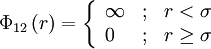$\Phi_{12}\left( r \right) = \left\{ \begin{array}{lll} \infty & ; & r < \sigma \\ 0 & ; & r \ge \sigma \end{array} \right.$

where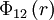$\Phi_{12}\left(r \right)$ is the intermolecular pair potential between two spheres at a distance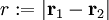$r := |\mathbf{r}_1 - \mathbf{r}_2|$, and$\sigma$ is the diameter of the sphere. The hard sphere model can be considered to be a special case of the hard ellipsoid model, where each of the semi-axes has the same length,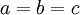$a=b=c$.

First simulations of hard spheres (1954-1957)

The hard sphere model, along with its two-dimensional manifestation hard disks, was one of the first ever systems studied using computer simulation techniques with a view to understanding the thermodynamics of the liquid and solid phases and their corresponding phase transition   , much of this work undertaken at the Los Alamos Scientific Laboratory on the world's first electronic digital computer ENIAC .

The following are a series of plots of the hard sphere radial distribution function  shown for different values of the number density$\rho$. The horizontal axis is in units of$\sigma$ where$\sigma$ is set to be 1. Click on image of interest to see a larger view.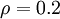$\rho=0.2$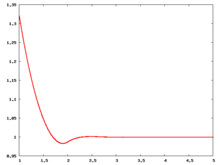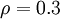$\rho=0.3$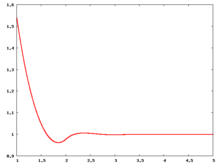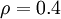$\rho=0.4$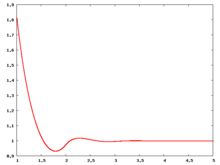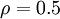$\rho=0.5$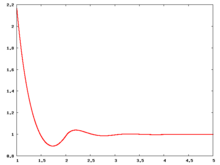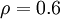$\rho=0.6$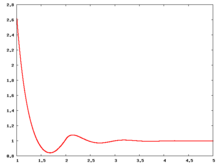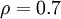$\rho=0.7$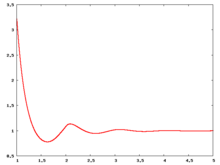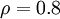$\rho=0.8$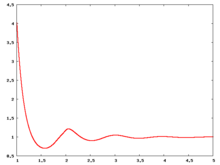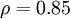$\rho=0.85$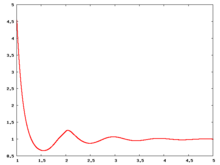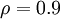$\rho=0.9$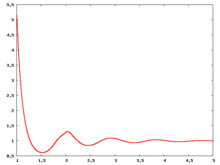The value of the radial distribution at contact,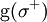${\mathrm g}(\sigma^+)$, can be used to calculate the pressure via the equation of state (Eq. 1 in )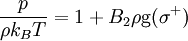$\frac{p}{\rho k_BT}= 1 + B_2 \rho {\mathrm g}(\sigma^+)$

where the second virial coefficient,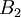$B_2$, is given by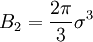$B_2 = \frac{2\pi}{3}\sigma^3$.

Carnahan and Starling  provided the following expression for${\mathrm g}(\sigma^+)$ (Eq. 3 in )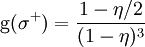${\mathrm g}(\sigma^+)= \frac{1-\eta/2}{(1-\eta)^3}$

where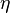$\eta$ is the packing fraction.

Over the years many groups have studied the radial distribution function of the hard sphere model:           

Liquid-solid transition

The hard sphere system undergoes a liquid-solid first order transition  , sometimes referred to as the Kirkwood-Alder transition . The liquid-solid coexistence densities (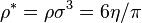$\rho^* = \rho \sigma^3=6\eta/\pi$) has been calculated to be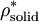$\rho^*_{\mathrm {solid}}$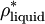$\rho^*_{\mathrm {liquid}}$ Reference 1.041 0.945  1.0376 0.9391  1.0367(10) 0.9387(10)  1.0372 0.9387  1.0369(33) 0.9375(14)  1.037 0.938  1.035(3) 0.936(2) 

The coexistence pressure has been calculated to be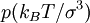$p (k_BT/\sigma^3)$ Reference 11.5727(10)  11.57(10)  11.567  11.55(11)  11.54(4)  11.50(9)  11.48(11)  11.43(17) 

The coexistence chemical potential has been calculated to be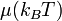$\mu (k_BT)$ Reference 15.980(11) 

The Helmholtz energy function (in units of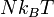$Nk_BT$) is given by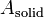$A_{\mathrm {solid}}$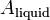$A_{\mathrm {liquid}}$ Reference 4.887(3) 3.719(8) 

The melting and crystallization process has been studied by Isobe and Krauth .

Helmholtz energy function

Values for the Helmholtz energy function ($A$) are given in the following Table: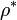$\rho^*$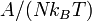$A/(Nk_BT)$ Reference 0.25 −1.766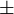$\pm$ 0.002 Table I  0.50 −0.152$\pm$ 0.002 Table I  0.75 1.721$\pm$ 0.002 Table I  1.04086 4.959 Table VI  1.099975 5.631 Table VI  1.150000 6.274 Table VI 

In  the free energies are given without the ideal gas contribution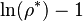$\ln(\rho^*)-1$ , hence it was added to the free energies in the table.

Interfacial Helmholtz energy function

The Helmholtz energy function of the solid–liquid interface has been calculated using the cleaving method giving (Ref.  Table I):

 work per unit area/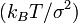$(k_BT/\sigma^2)$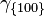$\gamma_{\{100\}}$ 0.5820(19)$\gamma_{\{100\}}$ 0.636(11)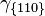$\gamma_{\{110\}}$ 0.5590(20)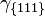$\gamma_{\{111\}}$ 0.5416(31)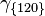$\gamma_{\{120\}}$ 0.5669(20)

Solid structure

The Kepler conjecture states that the optimal packing for three dimensional spheres is either cubic or hexagonal close packing, both of which have maximum densities of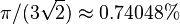$\pi/(3 \sqrt{2}) \approx 0.74048%$  . However, for hard spheres at close packing the face centred cubic phase is the more stable , with a Helmholtz energy function difference in the thermodynamic limit between the hexagonal close packed and face centered cubic crystals at close packing of 0.001164(8)$Nk_BT$. Recently evidence has been found for a metastable cI16 phase  indicating the "cI16 is a mechanically stable structure that can spontaneously emerge from a bcc starting point but it is thermodynamically metastable relative to fcc or hcp".

Direct correlation function

For the direct correlation function see:  

Bridge function

Details of the bridge function for hard sphere can be found in the following publication 

Equations of state

Main article: Equations of state for hard spheres

Virial coefficients

Main article: Hard sphere: virial coefficients

Experimental results

Pusey and van Megen used a suspension of PMMA particles of radius 305$\pm$10 nm, suspended in poly-12-hydroxystearic acid  For results obtained from the Colloidal Disorder - Order Transition (CDOT) experiments performed on-board the Space Shuttles Columbia and Discovery see Ref. 

Related systems

Hard spheres in other dimensions: